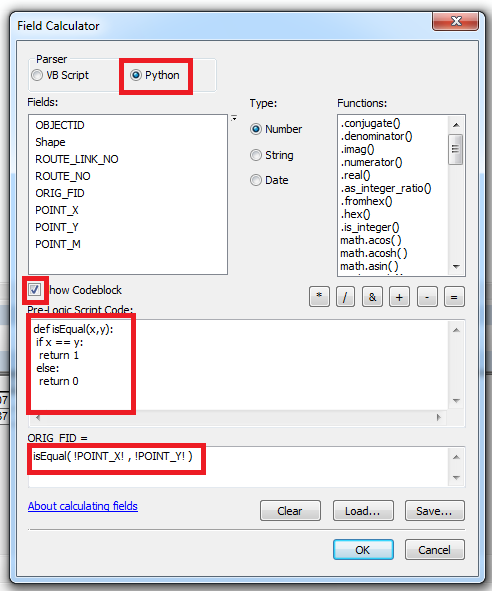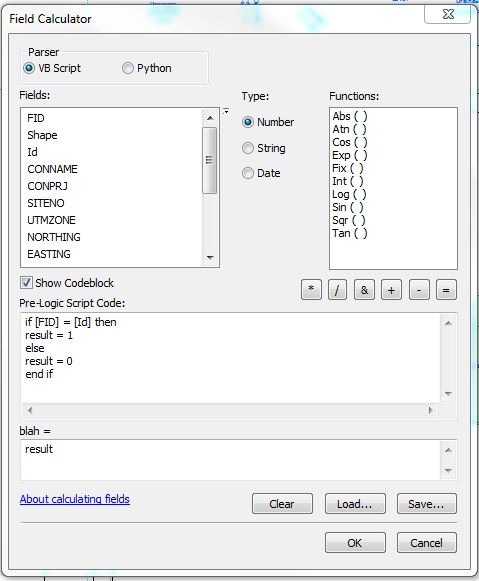# compare two fields if they 'are equal or not, in the field calculator

2847
4
08-20-2014 03:56 PMNew Contributor III

I have two "A" fields and "B" and I want to use the field calculator in the "C" field to determine if they are equal

put 1 if they are equal and 0 if they  not

example

A | B | C |

3 | 3 | 1 |

5 | 7 | 0 |

I found this scrip

but it only works in python window

import arcpy

fc = R 'c: \ path \ to \ your \ fc'

with arcpy.da.UpdateCursor (fc, ["FIELD1", "FIELD2", "FIELD3"]) as cursor:

for row in cursor:

if row  == row :

row  = 1

else:

row  = 0

cursor.updateRow (row)

"I want to use the field calculator"

or as a tool for modelbuidel where "fc = R'c: \ path \ to \ your \ fc '         can be a Shapefile imput

4 RepliesOccasional Contributor III

Hi Gustavo,

Try below:Script:

def isEqual(x,y):

if x == y:

return 1

else:

return 0byMVP Honored Contributor

Or in VBScript:New Contributor IIIby